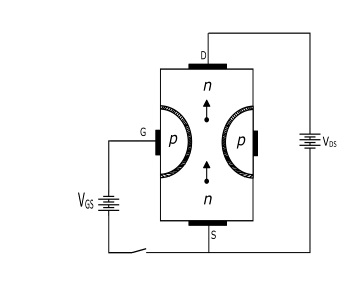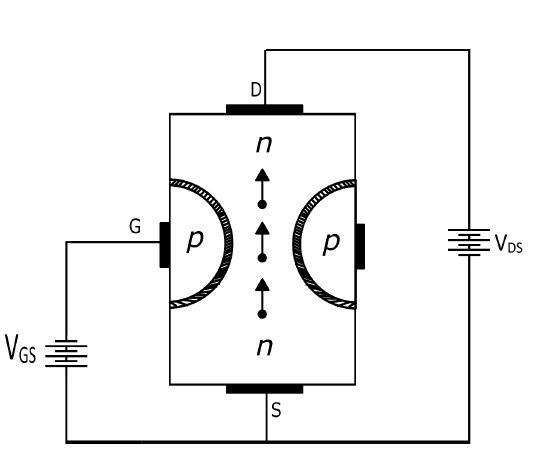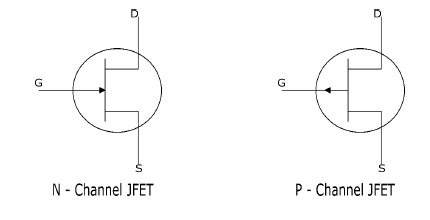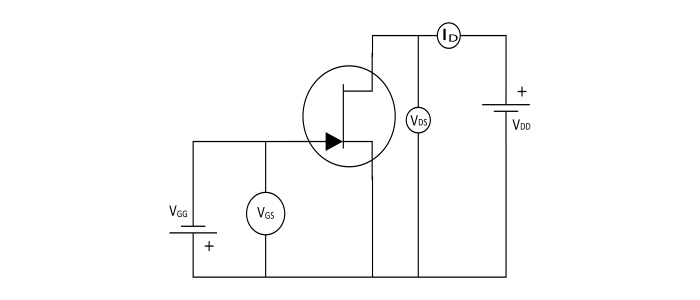# Junction Field Effect Transistor – Working Principle and Applications

Electronics & ElectricalDigital ElectronicsMiscellaneous

#### Ethical Hacking & Cyber Security Online Training

36 Lectures 5 hours

#### Machine Learning & BIG Data Analytics: Microsoft AZURE

46 Lectures 3.5 hours

#### Advance Big Data Analytics using Hive & Sqoop

Best Seller

50 Lectures 4 hours

The junction field effect transistor (JFET) is type of field effect transistor. It is a three terminal voltage controlled semiconductor device in which the current conduction is either by the electrons or holes. The current conduction is controlled by means of an electric field between the gate electrode and the conduction channel of the JFET.

## Construction of JFET

• N – Channel JFET −It consists of an n – type silicon bar forming the conduction channel for the charge carriers. The pn – junction forming diodes are connected internally and a common terminal called GATE is taken out from the p - Region. The other two terminals viz. Source and Drain are taken out from the bar.• P – Channel JFET − It consists of a p – type silicon bar forming the conduction channel for the charge carriers. The pn – junction forming diodes are connected internally and a common terminal called GATE is taken out from the n - Region. The other two terminals viz. Source and Drain are taken out from the bar.## Working Principle of JFET

When voltage VDS is applied between the drain and source terminals and gate terminal voltage is zero, the two pn-junctions at the sides establishes depletion layers. The electrons flow from source to drain through the channel between the depletion layers. The width of these depletion layers determine the width of the channel and hence the current conduction through the bar.Now, when a reverse voltage VGS is applied between the gate and the source terminals, the width of depletion layers is increased and this decreases the width of the conduction channel, thereby increasing the resistance of conduction channel. Consequently, the current from source to drain is decreased. On the other hand, when the reverse voltage VGS is decreased the width of depletion layer also decreases. Hence, the width of conduction channel increases and the resulting source to drain current.

Therefore, the current from source to drain can be controlled by the application voltage (electric field) on the gate terminal. For this reason it is known as Field Effect Transistor.

Hence, the JFET operates on the principle that the width and resistance of conduction channel can be varied by changing the reverse voltage VGS.### Schematic Symbol of JFET### Drain or Output Characteristics of JFETThe curve showing the relation between the drain current (ID) and the drain-source voltage (VDS) at constant gate-source voltage (VGS) is known as the Output or Drain Characteristics of JFET.

The important points from the output characteristics are −

• At the start, the drain current (ID) increases rapidly with increase in drain-source voltage (VDS) but then becomes constant. The value of VDS above which the drain current becomes constant is called as Pinch off Voltage (VP).

• After pinch off voltage, the channel width becomes so narrow that the depletion layers touch each other. Therefore, after pinch off voltage the change in the drain current is small with change in the VDS. Hence, the drain current remains constant.

• After pinch off voltage, the channel width becomes so narrow that the depletion layers touch each other. Therefore, after pinch off voltage the change in the drain current is small with change in the VDS. Hence, the drain current remains constant.

## Parameters of JFET

The JFET has parameters which determine the performance of it −

• AC drain resistance (rd) − It is defined as the ratio of the change in the drain-source voltage (ΔVDS) to the corresponding change in the drain current (ΔID) at constant gate-source voltage (VGS).

$$\mathrm{AC\:drain\:resistance(r_{d})=\frac{ΔV_{DS}}{ΔI_{D}}}$$

• Trans-conductance (gm) − It is defined as the ratio of change in drain current (ΔIDS) to the change in gate – source voltage (ΔVGS) at constant drain-source voltage.

The trans-conductance of JFET is expressed either in mA/V or microsiemens.

$$\mathrm{Transconductance(g_{m})=\frac{(ΔI_{D})}{(ΔV_{GS})}}$$

• Amplification Factor (μ) − It is defined as the ratio of change in drain-source voltage (ΔVDS) to the change in gate-source voltage (ΔGS) at constant drain current.

The amplification factor of JFET shows how much control the gate voltage has over the drain current.

$$\mathrm{Amplification\:Factor (\mu)=\frac{(ΔV_{DS})}{(ΔV_{GS})}}$$

## Advantages of JFET

• It has the high input impedance. This permits high degree of isolation between input-output circuit.

• JFET has a negative resistance temperature coefficient. This avoids the risk of thermal runaway.

• A JFET has a very high power gain.

• A JFET has smaller size, high efficiency and longer life.

## Applications of JFET

• A JFET can be used as a switch.
• JFET can be used as an amplifier.
• JFET can be used as a chopper.
• JFET can be used as a buffer.
• JFET can be used as voltage controlled resistors in the operational amplifiers.
• JFET is used in cascade amplifier and in RF amplifiers.
• JFET is used in communication devices.
• JFET is used in digital circuits.